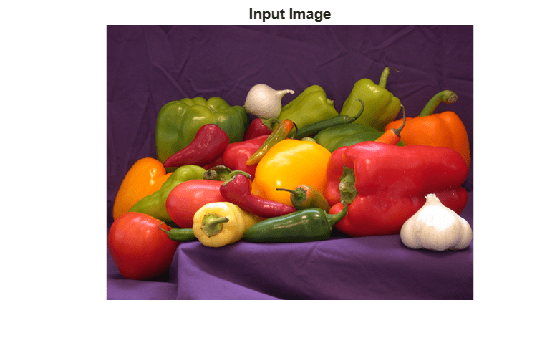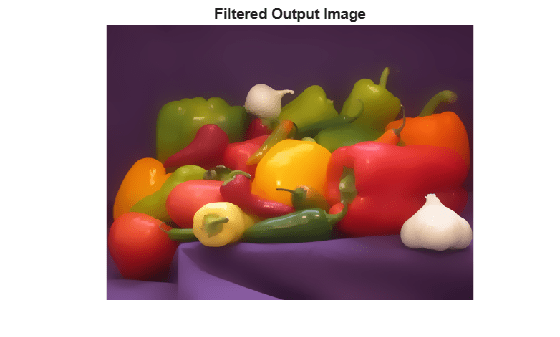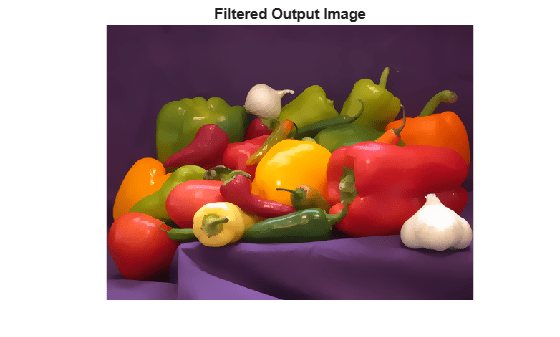# Perform Edge-Preserving Image Smoothing Using OpenCV in MATLAB

This example shows how to perform edge-preserving image smoothing by using prebuilt MATLAB® interface to the OpenCV function `cv::edgePreservingFilter`. In this example, you also use the `createMat` utility function to define the input and output arrays, and the `getImage` utility function to read the output image returned by the OpenCV function.

Add the MATLAB interface to OpenCV package names to the import list.

```import clib.opencv.*; import vision.opencv.util.*;```

Read an image into the MATLAB workspace.

`img = imread("peppers.png");`

Create MATLAB interface objects for the OpenCV `MatND` and `InputArray` classes to store the input image.

`[inputMat,inputArray] = createMat(img);`

Create MATLAB interface objects for the OpenCV `MatND` and `OutputArray` classes to write the output image returned by the OpenCV function.

`[outputMat,outputArray] = createMat;`

Smooth the input image by using the OpenCV function `cv::edgePreservingFilter`. To call this function from MATLAB, you must use the notation `cv.edgePreservingFilter`.

`cv.edgePreservingFilter(inputArray,outputArray);`

Read the filtered output image returned by the OpenCV function.

`filteredImg = getImage(outputArray);`

Display the original input and the filtered output images.

```figure imshow(img) title("Input Image")``````figure imshow(filteredImg) title("Filtered Output Image")```### Modify Filter Parameters

Specify the parameter values for the edge-preserving filter. Set these values:

• `flags` to `2`, to perform normalized convolution filtering.

• Standard deviations `sigma_s` to `20` and `sigma_r` to `0.3`.

```flags = 2; sigma_s = 20; sigma_r = 0.2;```

Perform filtering by using the defined filter parameters.

`cv.edgePreservingFilter(inputArray,outputArray,flags,sigma_s,sigma_r);`

Read the filtered output image returned by the OpenCV function.

`filteredImg = getImage(outputArray);`

Display the filtered output image.

```figure imshow(filteredImg) title("Filtered Output Image")```